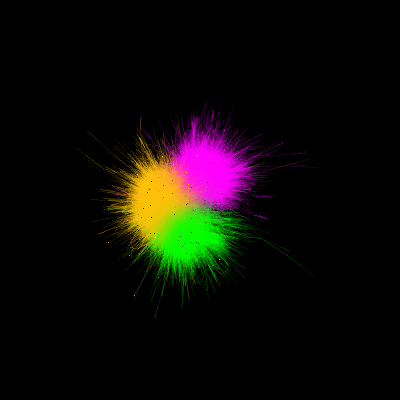# Streaming FURY with WebRTC/MJPEG#```webrtc not available
Dataset is already in place. If you want to fetch it again please first remove the folder /Users/skoudoro/.fury/examples/wiki_nw
```

```from os.path import join as pjoin
import multiprocessing
# if this example it's not working for you and you're using MacOs
# uncoment the following line
# multiprocessing.set_start_method('spawn')
import numpy as np
from fury import actor, window, colormap as cmap
from fury.stream.client import FuryStreamClient
from fury.data.fetcher import fetch_viz_wiki_nw
from fury.stream.server.main import web_server_raw_array

if __name__ == '__main__':

interactive = False
###########################################################################
# First we will set the resolution which it'll be used by the streamer

window_size = (400, 400)

files, folder = fetch_viz_wiki_nw()
categories_file, edges_file, positions_file = sorted(files.keys())
category2index = {
category: i
for i, category in enumerate(np.unique(categories))}

index2category = np.unique(categories)

categoryColors = cmap.distinguishable_colormap(
nb_colors=len(index2category))

colors = np.array([
categoryColors[category2index[category]]
for category in categories])

edgesPositions = []
edgesColors = []
for source, target in edges:
edgesPositions.append(np.array([positions[source], positions[target]]))
edgesColors.append(np.array([colors[source], colors[target]]))

edgesPositions = np.array(edgesPositions)
edgesColors = np.average(np.array(edgesColors), axis=1)

sphere_actor = actor.sdf(
centers=positions,
colors=colors,
primitives='sphere',

lines_actor = actor.line(
edgesPositions,
colors=edgesColors,
opacity=0.1,
)
scene = window.Scene()

scene.set_camera(
position=(0, 0, 1000),
focal_point=(0.0, 0.0, 0.0), view_up=(0.0, 0.0, 0.0))

showm = window.ShowManager(scene, reset_camera=False, size=(
window_size, window_size), order_transparent=False,
)

###########################################################################
# ms define the amount of mileseconds that will be used in the timer event.

ms = 0

stream = FuryStreamClient(
showm, use_raw_array=True)
p = multiprocessing.Process(
target=web_server_raw_array,
args=(
stream.img_manager.image_buffers,
stream.img_manager.info_buffer,
)
)
p.start()

stream.start(ms,)
if interactive:
showm.start()
stream.stop()
stream.cleanup()

window.record(
showm.scene, size=window_size, out_path="viz_no_interaction.png")
```

Total running time of the script: ( 0 minutes 2.362 seconds)

Gallery generated by Sphinx-Gallery